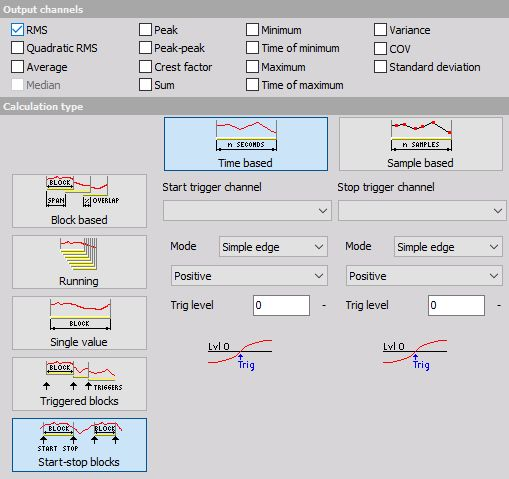# Basic statistics

When you press the Setup button on new Basic statistics line, the following Basic statistics setup window will open: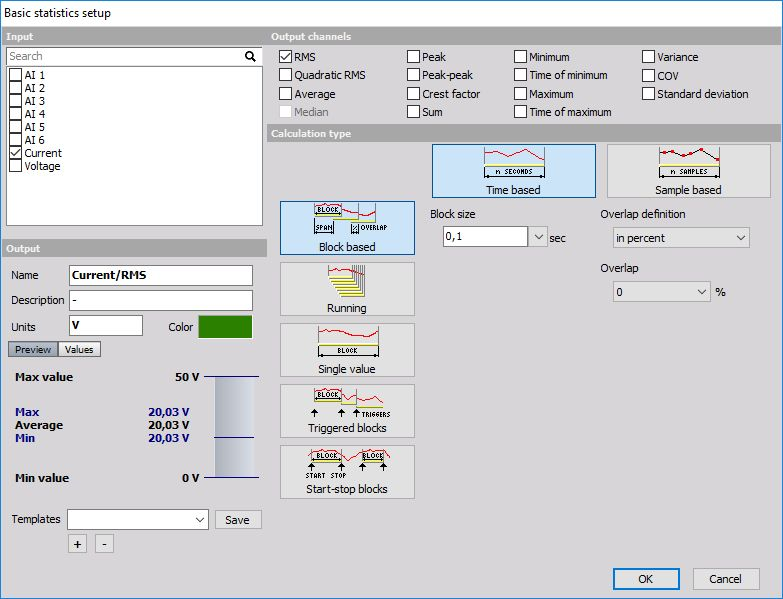Basic statistics setup window has a standard layout with Input channels in left top corner, Output information in left bottom and output statistics channels on right top. Right bottom is populated by different basic statistic calculation settings.

The statistics support multiple input channels.

For detailed information about basic settings of the input and output channels see  -> Setup screen and basic operation.

The statistic module provides basic statistical quantities of the signal. There are five basic calculation modes:

After choosing INPUT channel, we need to select statistical function on OUTPUT CHANNEL section.

## Output channel - Statistical functions

To select statistical function simply click (check) on the box beside its name on OUTPUT CHANNEL section: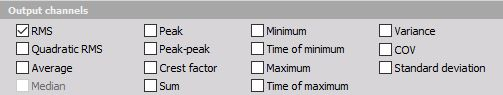• RMS - Will calculate the root mean square value of the signal
• Quadratic RMS - This is similar to the RMS, except all the values are double squared and summed.
• Average - Average will calculate the average or middle point.
• Median - Is the value separating the higher half of a data sample, from the lower half.
• Peak - Peak is the maximum deviation of the signal from the average value.
• Peak-peak - Is the difference between the minimum and maximum.
• Crest factor - Is the ratio between the peak and RMS value. Crest factor gives an impression about the spikes in the signal. Pure sine waves have a crest factor of 1.41.
• Sum - Sum of all acquired values in respect of the selected calculation type.
• Minimum - The minimum will calculate a minimum value of the signal for the specified period.
• Time of minimum - On the end of the block, it displays the time at which the minimum value occurred.
• Maximum - The maximum will calculate a maximum value of the signal for the specified period. This is very intensive operation and therefore unavailable in Running mode.
• Time of maximum - On the end of the block, it displays the time at which the maximum value occurred.
• Variance - Indicating how possible values of a signal are spread around the expected value.
• COV - Coefficient of variation is the normalized measure of dispersion of probability distribution. It is calculated as the ration between standard deviation and the mean.
• Standard deviation - The standard deviation is a measure of the spread of the values of the signal away from its mean, measuring how widely spread the values in a data set is. If the data points are close to the mean, then the standard deviation is small (if all the data values are equal, then the standard deviation is zero).

## Calculation type

### Block based

Block based calculation calculates the statistical quantity based on specific time interval defined by the block size.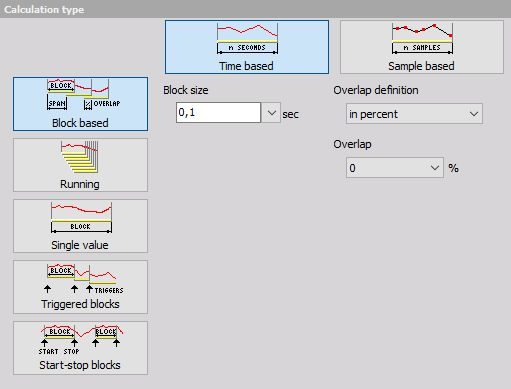• Block size in [sec] defines the time interval for calculation. 0,1 second in our case means that it will calculate the statistical quantities in 0,1 second interval. Therefore the resulting channels will have an update interval of 0,1 second.
• Overlap - Is useful when we need a specific time interval, but want to have a higher update rate of the resulting channels. In our case, the quantities will be updated in 0,1 second interval with 0% overlap and in 0,05 second interval with 50 % overlap. It means that the second block will not be calculated at the end of the first block, but half of the block before that. So the first block will be calculated from 0 to 0,1 second, second one from 0,05 to 0,15 second, third one from 0,1 to 0,2 second and so on.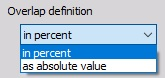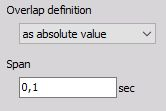Overlap can be defined:

### Running

Running calculation is an ‘extreme’ version of overlapping. The second block is calculated after one sample after the first block. Block size has the same meaning as for block based calculation.

With this method, we can only calculate RMS, Average, Quadratic RMS, Variance and Standard deviation statistical functions, because all others would be too intensive (especially minimum and maximum while all others relate to those two).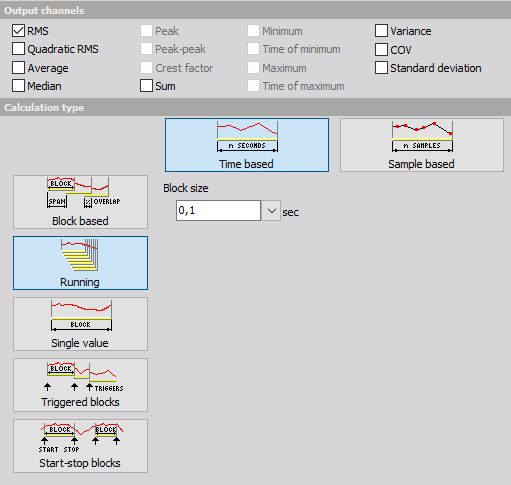### Single value

Single value is the simplest calculation and has no settings. It will produce only one value at the end of the measurement. The result will be updated also during the measurement, but only the final value will be stored in the data file.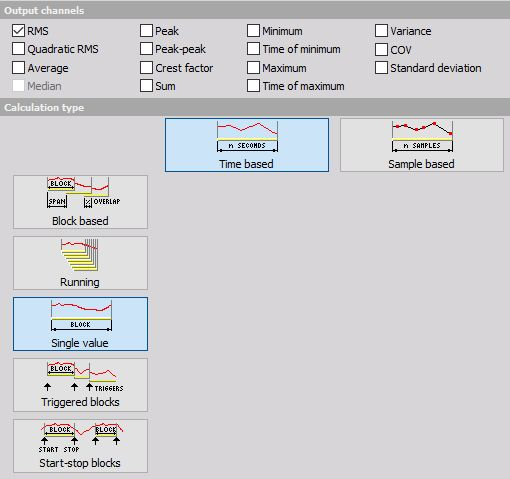### Triggered blocks

Triggered blocks option calculates the statistical value based on a specific trigger event. The calculation begins at the start of the acquisiton. When a trigger event is recognized, it stops the first calculation, writes the statistical value with its timestamp and then starts to calculate a new value. We can define any channel as the trigger channel and the settings for the trigger condition are the same as the alarm or storage triggers.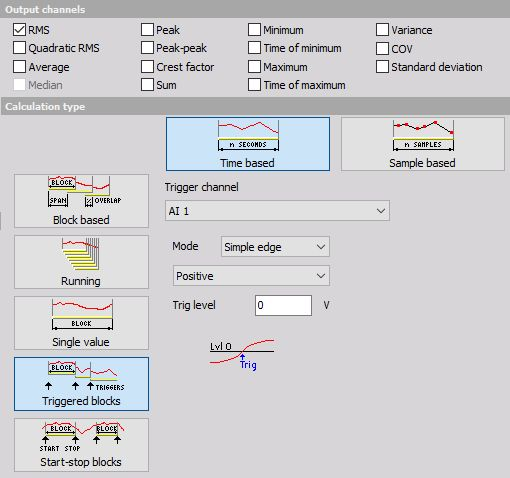### Start/stop blocks

Start/stop blocks option calculates the statistical value starting at a specific trigger event. When an event is recognized, it starts to calculate. When a stop condition is recognized, then the value is written to the resulting channel with the time stamp of stop event. It will wait with the calculation until the new start event is recognized. The start and stop channel can be any channel, also a different one and the trigger condition have the same options as the alarm or storage triggers.